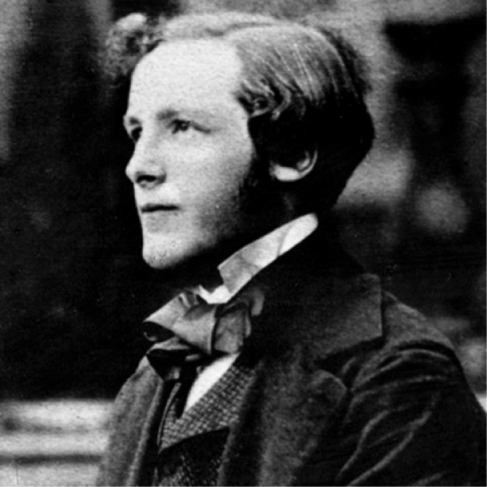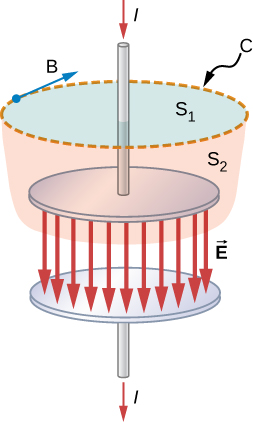16.1 Maxwell’s equations and electromagnetic waves

 Page 1 / 8
By the end of this section, you will be able to:
• Explain Maxwell’s correction of Ampère’s law by including the displacement current
• State and apply Maxwell’s equations in integral form
• Describe how the symmetry between changing electric and changing magnetic fields explains Maxwell’s prediction of electromagnetic waves
• Describe how Hertz confirmed Maxwell’s prediction of electromagnetic waves

James Clerk Maxwell (1831–1879) was one of the major contributors to physics in the nineteenth century ( [link] ). Although he died young, he made major contributions to the development of the kinetic theory of gases, to the understanding of color vision, and to the nature of Saturn’s rings. He is probably best known for having combined existing knowledge of the laws of electricity and of magnetism with insights of his own into a complete overarching electromagnetic theory, represented by Maxwell’s equations    .James Clerk Maxwell, a nineteenth-century physicist, developed a theory that explained the relationship between electricity and magnetism, and correctly predicted that visible light consists of electromagnetic waves.

Maxwell’s correction to the laws of electricity and magnetism

The four basic laws of electricity and magnetism had been discovered experimentally through the work of physicists such as Oersted, Coulomb, Gauss, and Faraday. Maxwell discovered logical inconsistencies in these earlier results and identified the incompleteness of Ampère’s law as their cause.

Recall that according to Ampère’s law, the integral of the magnetic field around a closed loop C is proportional to the current I passing through any surface whose boundary is loop C itself:

${\oint }_{C}\stackrel{\to }{B}·d\stackrel{\to }{s}={\mu }_{0}I.$

There are infinitely many surfaces that can be attached to any loop, and Ampère’s law stated in [link] is independent of the choice of surface.

Consider the set-up in [link] . A source of emf is abruptly connected across a parallel-plate capacitor so that a time-dependent current I develops in the wire. Suppose we apply Ampère’s law to loop C shown at a time before the capacitor is fully charged, so that $I\ne 0$ . Surface ${S}_{1}$ gives a nonzero value for the enclosed current I , whereas surface ${S}_{2}$ gives zero for the enclosed current because no current passes through it:

${\oint }_{C}\stackrel{\to }{B}·d\stackrel{\to }{s}=\left\{\begin{array}{ccc}{\mu }_{0}I\hfill & & \text{if surface}\phantom{\rule{0.2em}{0ex}}{S}_{1}\phantom{\rule{0.2em}{0ex}}\text{is used}\hfill \\ 0\hfill & & \text{if surface}\phantom{\rule{0.2em}{0ex}}{S}_{2}\phantom{\rule{0.2em}{0ex}}\text{is used}\hfill \end{array}.$

Clearly, Ampère’s law in its usual form does not work here. This may not be surprising, because Ampère’s law as applied in earlier chapters required a steady current, whereas the current in this experiment is changing with time and is not steady at all.The currents through surface S 1 and surface S 2 are unequal, despite having the same boundary loop C .

How can Ampère’s law be modified so that it works in all situations? Maxwell suggested including an additional contribution, called the displacement current ${I}_{\text{d}}$ , to the real current I ,

${\oint }_{C}\stackrel{\to }{B}·d\stackrel{\to }{s}={\mu }_{0}\left(I+{I}_{\text{d}}\right)$

where the displacement current is defined to be

${I}_{\text{d}}={\epsilon }_{0}\frac{d{\text{Φ}}_{\text{E}}}{dt}.$

Here ${\epsilon }_{0}$ is the permittivity of free space and ${\text{Φ}}_{\text{E}}$ is the electric flux    , defined as

${\text{Φ}}_{\text{E}}={\iint }_{\text{Surface}\phantom{\rule{0.2em}{0ex}}S}\stackrel{\to }{E}·d\stackrel{\to }{A}.$

The displacement current    is analogous to a real current in Ampère’s law, entering into Ampère’s law in the same way. It is produced, however, by a changing electric field. It accounts for a changing electric field producing a magnetic field, just as a real current does, but the displacement current can produce a magnetic field even where no real current is present. When this extra term is included, the modified Ampère’s law equation becomes

What is differential form of Gauss's law?
help me out on this question the permittivity of diamond is 1.46*10^-10.( a)what is the dielectric of diamond (b) what its susceptibility
a body is projected vertically upward of 30kmp/h how long will it take to reach a point 0.5km bellow e point of projection
i have to say. who cares. lol. why know that t all
Jeff
is this just a chat app about the openstax book?
kya ye b.sc ka hai agar haa to konsa part
what is charge quantization
it means that the total charge of a body will always be the integral multiples of basic unit charge ( e ) q = ne n : no of electrons or protons e : basic unit charge 1e = 1.602×10^-19
Riya
is the time quantized ? how ?
Mehmet
What do you meanby the statement,"Is the time quantized"
Mayowa
Can you give an explanation.
Mayowa
there are some comment on the time -quantized..
Mehmet
time is integer of the planck time, discrete..
Mehmet
planck time is travel in planck lenght of light..
Mehmet
it's says that charges does not occur in continuous form rather they are integral multiple of the elementary charge of an electron.
Tamoghna
it is just like bohr's theory. Which was angular momentum of electron is intral multiple of h/2π
determine absolute zero
The properties of a system during a reversible constant pressure non-flow process at P= 1.6bar, changes from constant volume of 0.3m³/kg at 20°C to a volume of 0.55m³/kg at 260°C. its constant pressure process is 3.205KJ/kg°C Determine: 1. Heat added, Work done, Change in Internal Energy and Change in Enthalpy
U can easily calculate work done by 2.303log(v2/v1)
Abhishek
Amount of heat added through q=ncv^delta t
Abhishek
Change in internal energy through q=Q-w
Abhishek
please how do dey get 5/9 in the conversion of Celsius and Fahrenheit
what is copper loss
this is the energy dissipated(usually in the form of heat energy) in conductors such as wires and coils due to the flow of current against the resistance of the material used in winding the coil.
Henry
it is the work done in moving a charge to a point from infinity against electric field
what is the weight of the earth in space
As w=mg where m is mass and g is gravitational force... Now if we consider the earth is in gravitational pull of sun we have to use the value of "g" of sun, so we can find the weight of eaeth in sun with reference to sun...
Prince
g is not gravitacional forcé, is acceleration of gravity of earth and is assumed constante. the "sun g" can not be constant and you should use Newton gravity forcé. by the way its not the "weight" the physical quantity that matters, is the mass
Jorge
Yeah got it... Earth and moon have specific value of g... But in case of sun ☀ it is just a huge sphere of gas...
Prince
Thats why it can't have a constant value of g ....
Prince
not true. you must know Newton gravity Law . even a cloud of gas it has mass thats al matters. and the distsnce from the center of mass of the cloud and the center of the mass of the earth
Jorge
please why is the first law of thermodynamics greater than the second
every law is important, but first law is conservation of energy, this state is the basic in physics, in this case first law is more important than other laws..
Mehmet
First Law describes o energy is changed from one form to another but not destroyed, but that second Law talk about entropy of a system increasing gradually
Mayowa
first law describes not destroyer energy to changed the form, but second law describes the fluid drection that is entropy. in this case first law is more basic accorging to me...
Mehmet
define electric image.obtain expression for electric intensity at any point on earthed conducting infinite plane due to a point charge Q placed at a distance D from it.
explain the lack of symmetry in the field of the parallel capacitor
pls. explain the lack of symmetry in the field of the parallel capacitor
PhoebeByByBy RhodesByBy Prateek Ashtikar